# Little bit of History

It was created by John McCarthy in 1958, as a mathematical notation for computer programs.

Was not intended to be an actual programming language... until someone (Steve Russell) wrote an interpreter for it.

# There are many LISPs

Lisp is a family of computer programming languages:

• Common Lisp
• Dylan
• Scheme
• Clojure
• Arc
• AutoLisp
• ....

# There are many LISPs

We will concentrate in one of them:

Common Lisp

# Lisp is Different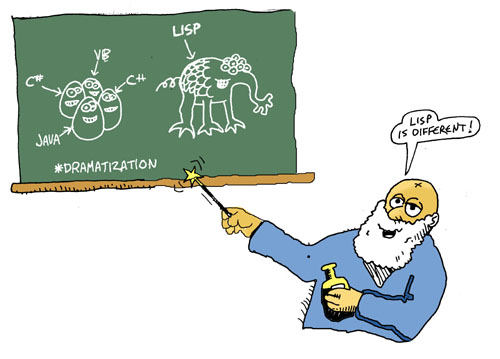http://www.lisperati.com/casting.html

# Lisp is Different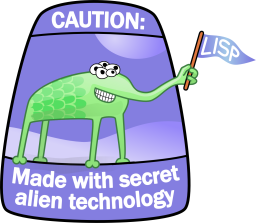# Syntax

A Lisp expression (also called form) can be:

• An atom: `2, 3, hello, "world"`
• A list of atoms and other lists: `(1 2 4 (5 6) 7)`
• An empty list: `()` which is also represented by the atom `nil`

# Atoms

Atoms are only separated by whitespace and lists

```23
hello!!
@I'm-a-whole-atom
/Me_too/
```

# Lists

Lists can be nested.

```(a b (c d) e (f (g h)))
((()))
((10 a) "no")
```

# Evaluation

Atoms can represent many things, most commonly numbers, strings, and symbols.

Numbers and strings evaluate to themselves:

```1
=> 1

"hello"
=> "hello"
```

# Evaluation

A symbol is an atom that can have a value,

```hello
=> ERROR: The variable HELLO is unboud.

(defparameter hello "Hello World!")

hello
=> "Hello World!"
```

# Evaluation

A function application f(x) is written as `(f x)`

This is prefix notation.

`(function arg1 arg2 arg3 ....)`

For example:

```(+ 1 2 3)
=> 6

(print "Foo")
=> "Foo"
```

# Evaluation

Lisp evaluates the arguments (left to right), then applies the function (the first element of the list) to these arguments.

# Evaluation

Defining functions

```(defun factorial (x)
(if (eql x 0)
1
(* x (factorial (- x 1)))))

(factorial 5)
=> 120

(factorial (+ 2 3))
=> 120
```

# Special Forms

There are "Special Forms" and "Macros", that look like regular functions but don't obey the argument evaluation rules defined for functions.

# Special Forms

The special form `QUOTE` prevents evaluation of its argument.

```(print hello)
=> "Hello World!"

(print (quote hello))
=> HELLO
```

# Special Forms

There's a shortcut for `QUOTE` which is the apostrophe `'`

```(print 'hello)
=> HELLO

(print 'hey-im-a-random-symbol)
=> HEY-IM-A-RANDOM-SYMBOL
```

# Special Forms

Another special form is `IF`

```(if (equal 20 (+ 15 5))
(print "I know how to sum!")
(print "I failed basic math..."))

=> "I know how to sum!"
```

# Special Forms

There are three arguments to the previous `IF` example:

• (equal 20 (+ 15 5))
• (print "I know how to sum!")
• (print "I failed basic math...")

# Special Forms

The first argument is evaluated:

```(equal 20 (+ 15 5))
=> T
```

And if the result is a true value (which the symbol `T` is), the second argument to `IF` is evaluated, otherwise it is the third argument that is evaluated.

# Special Forms

There is no difference between expressions and statements

All forms return a value!

Always...

```(print (if (> 5 3) "Five" "What??"))
=> "Five"
```

# Special Forms

`LET` defines values for symbols, but these symbols are only valid within the scope of `LET`.

```(let ((a 3)
(b 4)
(c 5))
(* (+ a b) c))
=> 35
```

`LET` looks like: `(let (bindings) forms)`.

# Special Forms

`COND` lets us evaluate forms conditionally:

```(defun is-my-favorite-number? (num)
(cond ((equal num 10) "Yes it is")
((evenp num) "I like even numbers")
(t "Nope, try with another one")))

(is-my-favorite-number? 10)
=> "Yes it is"

(is-my-favorite-number? 2)
=> "I like even numbers"

(is-my-favorite-number? 3)
=> "Nope, try with another one"
```

# Special Forms

We can change values of bindings with `SETF`:

```hello
=> "Hello World!"

(setf hello "Hola Mundo!")
=> "Hola Mundo!"

hello
=> "Hola Mundo!"
```

# Special Forms

We can change values of bindings with `SETF`:

```(let ((name "Jorge"))
(format t "My name is ~a~%" name)
(setf name "George")
(format t "Or ~a if you'd like." name))

My name is Jorge
Or George if you'd like.
=> NIL
```

# Creating Lists

`CONS` is the basic constructor of lists:

```(cons 1 nil)
=> (1)

(cons 1 (cons 2 (cons 3 nil)))
=> (1 2 3)

(cons 'hey (cons "you" nil))
=> (HEY "you")
```

# Creating Lists

It's easier to create lists with `LIST`:

```(list 1 2 3)
=> (1 2 3)

(list 'hey "you" 10)
=> (HEY "you" 10)

(list 'a (list 'b 'c) 2 3)
=> (A (B C) 2 3)
```

# CONS Cells

Lisp uses a primitive object to create lists, the Cons Cell.

A Cons Cell is an object with two slots, which for historical reasons, are called the `CAR` and `CDR`

# CONS Cells

Remember that:

```(cons 1 (cons 2 (cons 3 nil)))
=> (1 2 3)
```

A picture of the cons cells is:From: http://www.gigamonkeys.com/book/

# CONS Cells

```(list "foo" (list 1 2) 10)
=> ("foo" (1 2) 10)
```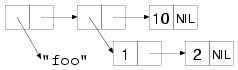From: http://www.gigamonkeys.com/book/

# Lists

You can use lists to represent trees of any depth and complexity: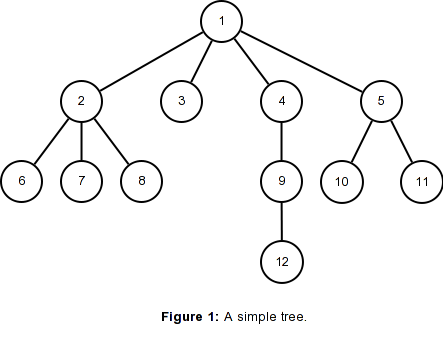# Lists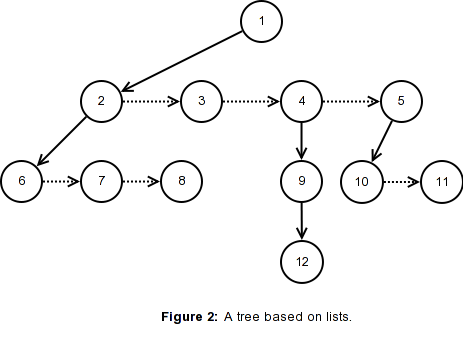# Lists`(1 2 3 4 5)`

# Lists`(1 (2 6 7 8) 3 4 5)`

# Lists`(1 (2 6 7 8) 3 (4 9) 5)`

# Lists`(1 (2 6 7 8) 3 (4 (9 12)) 5)`

# Lists`(1 (2 6 7 8) 3 (4 (9 12)) (5 10 11))`

# Lists`(1 (2 6 7 8) 3 (4 (9 12)) (5 10 11))`

# CAR and CDR

You can use the functions `CAR` and `CDR` to access the contents of the two slots of a cons cell.

```(let ((mylist (list 1 2 3)))
(format t "First element: ~a~%" (car mylist))
(format t "The rest: ~a~%" (cdr mylist)))

First element: 1
The rest: (2 3)
=> NIL
```# CAR and CDR

There are nicknames for `CAR` and `CDR`, called `FIRST` and `REST`

```(let ((mylist (list 1 2 3)))
(format t "First element: ~a~%" (first mylist))
(format t "The rest: ~a~%" (rest mylist)))

First element: 1
The rest: (2 3)
=> NIL
```# CAR and CDR

But lispers usually stick to `CAR` and `CDR`

The advantage is that they can be combined:

```(format nil "Second element is ~a~%" (cadr (list 1 2 3)))
=> "Second element is 2"
```

# CAR and CDR

```(let ((foo '((three 4) (five (6)) "seven" 8)))

=> (4 (FIVE (6)) "seven" 8)
```

# Multiple Values

A function can return more than one value, for example the function `FLOOR` divide a number by a divisor and returns two values, the quotient and remainder.

```(floor 20 2)
=> 10
=> 0

(floor 20 3)
=> 6
=> 2
```

# Multiple Values

Normally we only access the first value:

```(equal 6 (floor 20 3))
=> T

(multiple-value-bind (quotient remainder) (floor 20 3)
(format t "The quot is ~a and the rem is ~a~%" quotient remainder)
(+ (* quotient 3) remainder))

The quot is 6 and the rem is 2
=> 20
```

# Multiple Values

We can return multiple values from our functions with the `VALUES` function:

```(defun foo (x)
(values
(* 2 x)
(* 3 x)))

(multiple-value-bind (twotimes threetimes) (foo 4)
(list twotimes threetimes))
=> (8 12)
```

# Data Types: Numbers

Common Lisp has a rich number type system: Reals, Integers, Rationals, Fixnums, Bignums, and Complex.

```10.23
=> 10.23

(/ 1 3)
=> 1/3

(+ 2/3 1/3)
=> 1

(+ 2/3 5/3)
=> 7/3
```

# Data Types: Numbers

Common Lisp has a rich number type system: Reals, Integers, Rationals, Fixnums, Bignums, and Complex.

```#c(10 2)
=> #C(10 2)

(expt 2 1000)
=> 10715086071862673209484250490600018105614048117055336074437503883703510511249361224931983788156958581275946729175531468251871452856923140435984577574698574803934567774824230985421074605062371141877954182153046474983581941267398767559165543946077062914571196477686542167660429831652624386837205668069376 ```

# Arrays

```(defparameter arr (make-array '(2 3) :initial-element nil))
=> #2A((NIL NIL NIL) (NIL NIL NIL))

(aref arr 0 0)
=> NIL

(setf (aref arr 0 0) 'b)
=> B

arr
=> #2A((B NIL NIL) (NIL NIL NIL))
```

# Arrays

```(setf (aref arr 1 0) 'c
(aref arr 1 1) 'd)

arr
=> #2A((B NIL NIL) (C D NIL))
```

# Vectors

Vectors are one-dimensional arrays:

```(defparameter v1 (make-array '(3)))

(setf (aref v1 0) 'zero
(aref v1 1) 'one)

v1
=> #(ZERO ONE 0)
```

# Hash Tables

A Hash Table associates values with keys:

```(setq ht1 (make-hash-table))

(gethash 'quux ht1)
=> NIL
=> NIL

(setf (gethash 'baz ht1) 'baz-value)
(gethash 'baz ht1)
=> BAZ-VALUE
=> T

(setf (gethash 'gronk ht1) nil)
(gethash 'gronk ht1)
=> NIL
=> T```

# Looping

```(dotimes (i 5)
(format t "Number: ~a~%" (+ 2 i)))

Number: 2
Number: 3
Number: 4
Number: 5
Number: 6
=> NIL
```

# The Loop Macro

```(loop for i from 1 to 10 collecting i)
=> (1 2 3 4 5 6 7 8 9 10)

(loop for x from 1 to 10 summing (expt x 2))
=> 385

(loop for x across "the quick brown fox jumps over the lazy dog"
counting (find x "aeiou"))
=> 11```

# The Loop Macro

```(loop for x in '(1 2 3 4)
collect (1+ x))
=> (2 3 4 5)
```

# The Loop Macro

```(defun even/odd (ns)
(loop for n in ns
if (evenp n)
collect n into evens
else collect n into odds
finally (return (values evens odds))))

(even/odd (list 1 2 3 5 6 8 11 20 54))
=> (2 6 8 20 54)
=> (1 3 5 11)
```

# The Loop Macro

```(defparameter *random* (loop repeat 100 collect (random 10000)))

(loop for i in *random*
counting (evenp i) into evens
counting (oddp i) into odds
summing i into total
maximizing i into max
minimizing i into min
finally (return (list min max total evens odds)))
=> (69 9918 479477 55 45)
```

# Anonymous functions

`LAMBDA` can be used to define functions without giving them an explicit name:

```(defun double-me (x) (* 2 x))

(double-me 4)
=> 8

((lambda (x) (* 2 x)) 4)
=> 8```

# MAPCAR

`MAPCAR` traverses a list and applies a function to each element of the list, returning a new list with the results:

```(mapcar #'double-me (list 1 2 3 4))
=> (2 4 6 8)

(mapcar (lambda (x) (* 3 x)) (list 1 2 3 4))
=> (3 6 9 12)```

# Functions are first class objects

```(defun curry (fn &rest args)
(lambda (&rest rest)
(apply fn (append args rest))))

(funcall (curry #'* 2) 10)
=> 20

(mapcar (curry #'+ 10) (list 1 2 6 7 30 40))
=> (11 12 16 17 40 50)
```

# REDUCE

```(reduce #'+ #(1 2 3 4 5 6 7 8 9 10))
=> 55
```

# Macros

A Macro lets us do transformations to our source code before it's even compiled.

They look a lot like functions, the form a macro definition is:

`(defmacro name (arguments) body)`

# Macros

```(defmacro reverse-cons (rest first)
`(cons ,first ,rest))

(reverse-cons nil 2)
=> (2)```

# Macros

```(defmacro my-when (condition &rest body)
`(if ,condition (progn ,@body)))

(my-when (> 5 2)
;; do something...
'blah)
=> BLAH
```

# Macros

```(defmacro my-unless (condition &rest body)
`(if (not ,condition) (progn ,@body)))

(my-unless (= 5 2)
(print "Of course it is not the same"))
=> "Of course it is not the same"
```

# Macros

```(defmacro aif (test then &optional else)
`(let ((it ,test))
(if it ,then ,else)))

(aif (get-customer "Pepe")
(print (customer-name it))
=> "Pepito"

(aif (get-customer "Foo")
(print (customer-name it))
```

# More functional functions

```(defun memoize (fn)10) (list 1 2 6 7 30 40))
(let ((cache (make-hash-table :test #'equal)))
(lambda (&rest args)
(aif (gethash args cache)
it
(setf (gethash args cache) (apply fn args))))))
```

# More functional functions

```(defun expensive-func (x)
(sleep x)
(* 2 x))

(defparameter *memoized-expensive-func* (memoize #'expensive-func))

(funcall *memoized-expensive-func* 3)
=> 6  ;This function call takes 3 seconds to complete.

(funcall *memoized-expensive-func* 3)
=> 6  ;This function call returns immediately```

# Common Lisp Object System (CLOS)

```(defclass rectangle ()
((height :initarg :start-height
:accessor rectangle-height)
(width :initarg :start-width
:accessor rectangle-width)))
```

# Common Lisp Object System (CLOS)

```(defparameter r1 (make-instance 'rectangle
:start-height 50
:start-width 100))

(rectangle-height r1)
=> 50

(rectangle-width r1)
=> 100
```

# Common Lisp Object System (CLOS)

```(defclass color-rectangle (rectangle)
((color :initarg :color
:accessor rectangle-color)))
```

# Common Lisp Object System (CLOS)

```(defparameter r2 (make-instance 'color-rectangle
:start-height 10
:start-width 15
:color 'red))

(format nil "A ~a rectangle of ~ax~a"
(rectangle-color r2)
(rectangle-height r2)
(rectangle-width r2))
=> "A RED rectangle of 10x15"
```

# Common Lisp Object System (CLOS)

```(defclass circle ()
```

# Common Lisp Object System (CLOS)

```(defclass output-medium ()
((name :initarg :name
:accessor medium-name)))

(defclass screen (output-medium)
((res-x :initarg :res-x
:accessor screen-res-x)
(res-y :initarg :res-y
:accessor screen-res-y)))

(defclass printer (output-medium)
((location :initarg :location
:accessor printer-location)))
```

# Common Lisp Object System (CLOS)

```(defgeneric paint (shape medium))

(defmethod paint ((shape rectangle) medium)
(format nil "Drawing a rectangle of ~ax~a, on unkown medium ~a"
(rectangle-height shape)
(rectangle-width shape)
(medium-name medium)))

(defmethod paint ((shape circle) medium)
(format nil "Drawing a circle or radius ~a, on unknown medium ~a"
(medium-name medium)))
```

# Common Lisp Object System (CLOS)

```(defmethod paint ((shape rectangle) (medium screen))
(format nil "Drawing a rectangle of ~ax~a,
on screen ~a of ~a by ~a pixels"
(rectangle-height shape)
(rectangle-width shape)
(medium-name medium)
(screen-res-x medium)
(screen-res-y medium)))
```

# Common Lisp Object System (CLOS)

```(defmethod paint ((shape rectangle) (medium printer))
(format nil "Drawing a rectangle of ~ax~a,
on printer ~a located at ~a"
(rectangle-height shape)
(rectangle-width shape)
(medium-name medium)
(printer-location medium)))
```

# Common Lisp Object System (CLOS)

```(let ((r1 (make-instance 'rectangle
:start-height 15
:start-width 20))
(plotter (make-instance 'output-medium :name "Plotter"))
(screen1 (make-instance 'screen
:name "Big Screen"
:res-x 1024 :res-y 780))
(printer1 (make-instance 'printer
:name "HP blah"
:location "downstairs")))
(list
(paint r1 plotter)
(paint r1 screen1)
(paint r1 printer1)
(paint c1 plotter)))
```

# Common Lisp Object System (CLOS)

```=> ("Drawing a rectangle of 15x20, on unkown medium Plotter"
"Drawing a rectangle of 15x20,
on screen Big Screen of 1024 by 780 pixels"
"Drawing a rectangle of 15x20,
on printer HP blah located at downstairs"
"Drawing a circle or radius 30, on unknown medium Plotter")
```